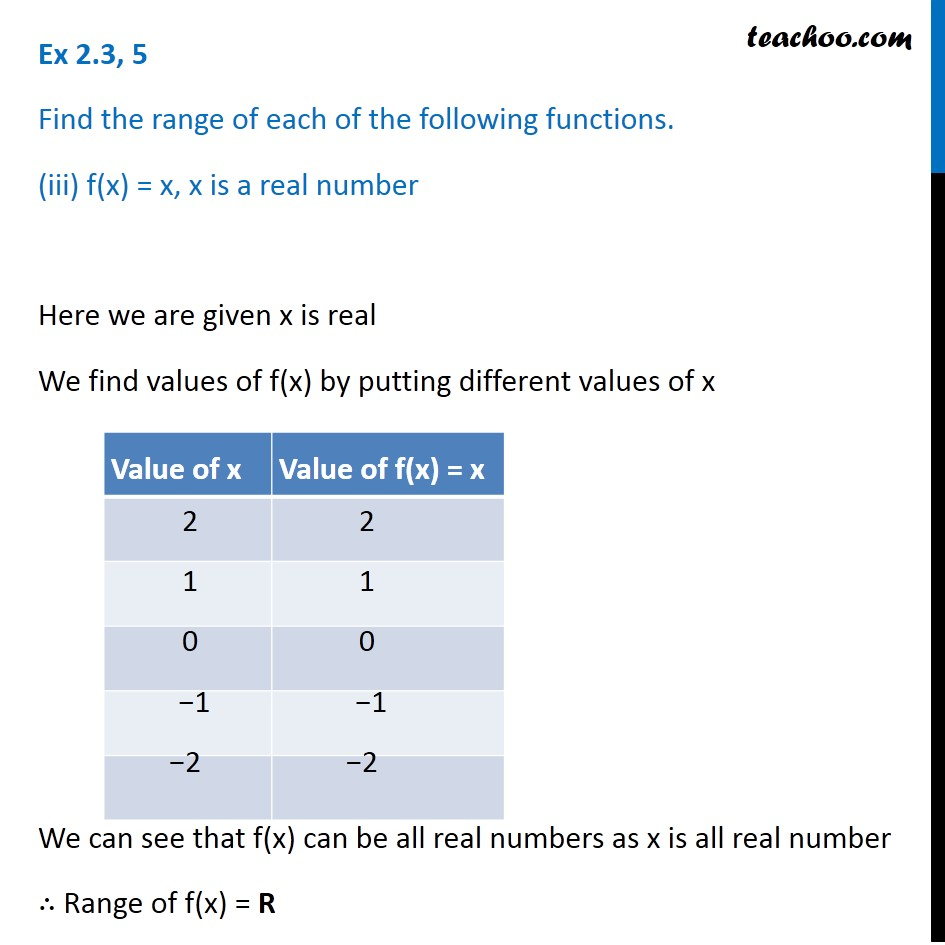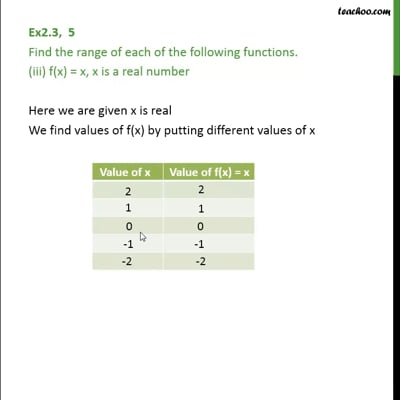Ex 2.3

Chapter 2 Class 11 Relations and Functions
Serial order wiseThis video is only available for Teachoo black users

Learn in your speed, with individual attention - Teachoo Maths 1-on-1 Class

### Transcript

Ex 2.3, 5 Find the range of each of the following functions. (iii) f(x) = x, x is a real number Here we are given x is real We find values of f(x) by putting different values of x We can see that f(x) can be all real numbers as x is all real number ∴ Range of f(x) = R